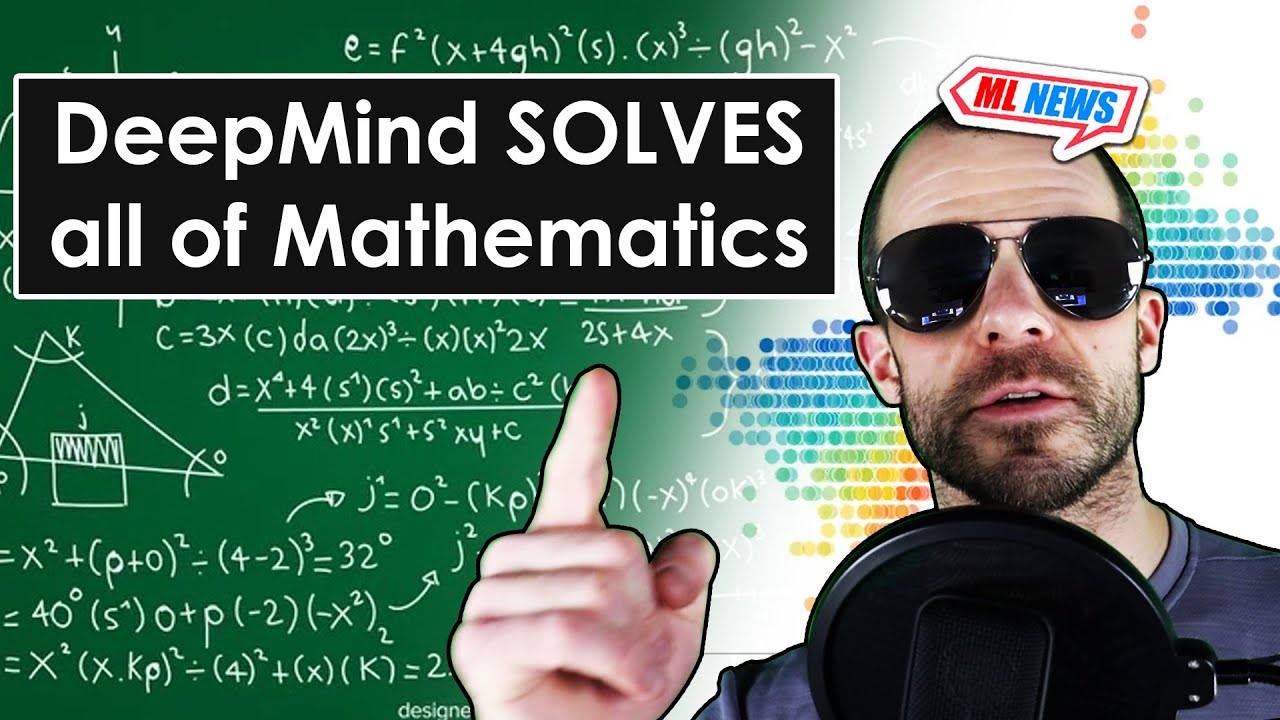# deepmind mathAI Mathematics

#### This is a game changer! (AlphaTensor by DeepMind explained)

Matrix multiplication is the most used mathematical operation in all of science and engineering. Speeding this up has massive consequences. Thus, over the years, this operation has become more and more optimized. A fascinating discovery was made when it was shown that one actually needs less than N^3 multiplication operations to multiply to NxN matrices. […]